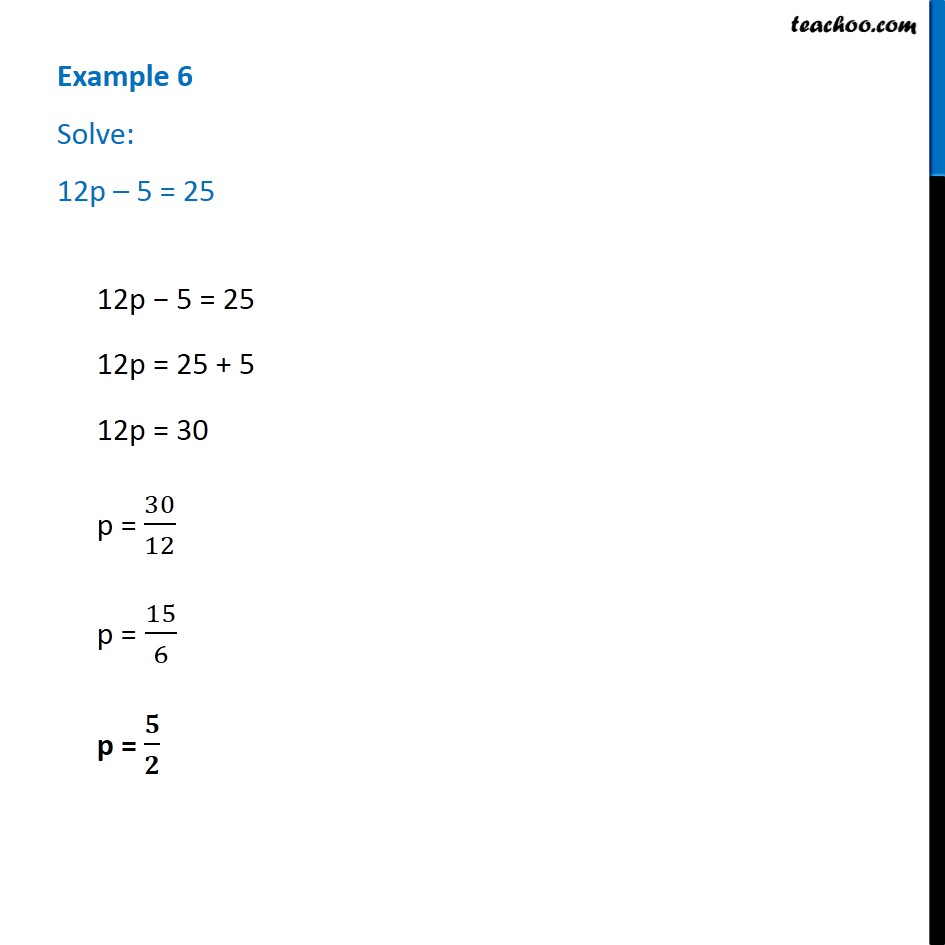1. Chapter 4 Class 7 Simple Equations
2. Concept wise
3. Solving Equations

Transcript

Example 6 - Chapter 4 Class 7 Simple Equations - NCERT Books Solve: 12p – 5 = 25 12p − 5 = 25 12p = 25 + 5 12p = 30 p = 30/12 p = 15/6 p = 5/2

Solving Equations